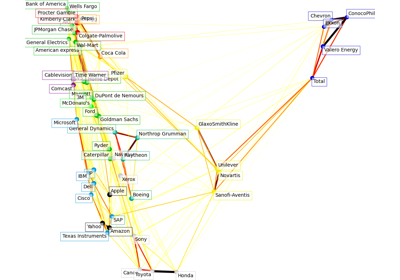# sklearn.cluster.affinity_propagation¶

sklearn.cluster.affinity_propagation(S, *, preference=None, convergence_iter=15, max_iter=200, damping=0.5, copy=True, verbose=False, return_n_iter=False, random_state='warn')[source]

Perform Affinity Propagation Clustering of data

Read more in the User Guide.

Parameters
Sarray-like, shape (n_samples, n_samples)

Matrix of similarities between points

preferencearray-like, shape (n_samples,) or float, optional

Preferences for each point - points with larger values of preferences are more likely to be chosen as exemplars. The number of exemplars, i.e. of clusters, is influenced by the input preferences value. If the preferences are not passed as arguments, they will be set to the median of the input similarities (resulting in a moderate number of clusters). For a smaller amount of clusters, this can be set to the minimum value of the similarities.

convergence_iterint, optional, default: 15

Number of iterations with no change in the number of estimated clusters that stops the convergence.

max_iterint, optional, default: 200

Maximum number of iterations

dampingfloat, optional, default: 0.5

Damping factor between 0.5 and 1.

copyboolean, optional, default: True

If copy is False, the affinity matrix is modified inplace by the algorithm, for memory efficiency

verboseboolean, optional, default: False

The verbosity level

return_n_iterbool, default False

Whether or not to return the number of iterations.

random_stateint or np.random.RandomStateInstance, default: 0

Pseudo-random number generator to control the starting state. Use an int for reproducible results across function calls. See the Glossary.

New in version 0.23: this parameter was previously hardcoded as 0.

Returns
cluster_centers_indicesarray, shape (n_clusters,)

index of clusters centers

labelsarray, shape (n_samples,)

cluster labels for each point

n_iterint

number of iterations run. Returned only if return_n_iter is set to True.

Notes

For an example, see examples/cluster/plot_affinity_propagation.py.

When the algorithm does not converge, it returns an empty array as cluster_center_indices and -1 as label for each training sample.

When all training samples have equal similarities and equal preferences, the assignment of cluster centers and labels depends on the preference. If the preference is smaller than the similarities, a single cluster center and label 0 for every sample will be returned. Otherwise, every training sample becomes its own cluster center and is assigned a unique label.

References

Brendan J. Frey and Delbert Dueck, “Clustering by Passing Messages Between Data Points”, Science Feb. 2007

## Examples using sklearn.cluster.affinity_propagation¶